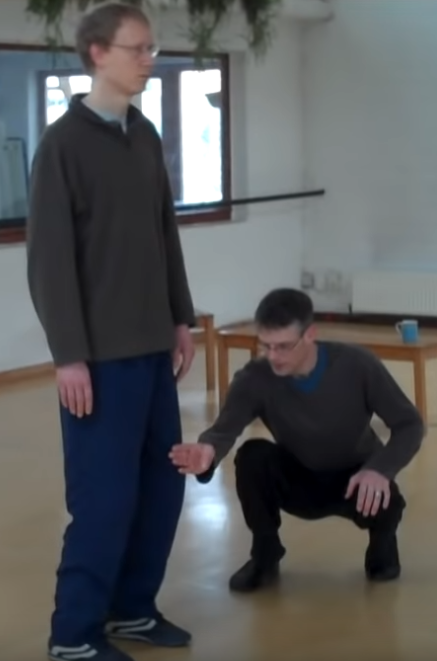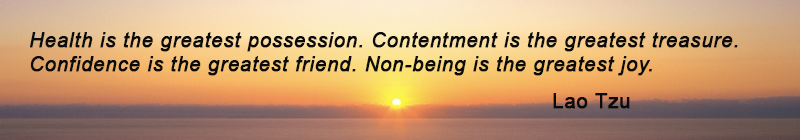Like   Tweet   Pin   +1   in/* styles */ Tips & resources on wellbeing & performance   ||   Issue 36, October 2021
 table div table+table+table div table{width:100%;padding:0}table div table+table+table div table img{width:96.23%;padding:0;float:none}table div table+table+table div table td{width:100%;padding:0 1.88% 18px}/* styles */In this issue there are tips on relaxation, concentration, hip alignment working with residents in aged care. And the usual inspiring Lao Tzu quote - this one on the three greatest things a person can have.

Regards
Chris Bennett
Chris Chi

 table div table+table+table+table+table div table{width:100%;padding:0}table div table+table+table+table+table div table img{width:96.23%;padding:0;float:none}table div table+table+table+table+table div table td{width:100%;padding:0 1.88% 18px}/* styles */## Deep Relaxation

 /* styles */ Here's one exercise I do as part of my Tai Chi training that is very relaxing. I lie on my carpeted floor, face up, legs slightly apart, arms beside my body, palms down. I use a yoga mat for extra comfort and place a thick folded towel under my head to keep my neck straight. I focus on my breathing, eyes closed, and imagine I'm gently sinking into the ground. I plan to use a timer in future because I almost fell asleep the other day!
 table div table+table+table+table+table+table+table+table div table{width:100%;padding:0}table div table+table+table+table+table+table+table+table div table img{width:96.23%;padding:0;float:none}table div table+table+table+table+table+table+table+table div table td{width:100%;padding:0 1.88% 18px}/* styles */## Tai Chi Tip

 table div table+table+table+table+table+table+table+table+table+table+table div table{width:100%;padding:0}table div table+table+table+table+table+table+table+table+table+table+table div table img{width:96.23%;padding:0;float:none}table div table+table+table+table+table+table+table+table+table+table+table div table td{width:100%;padding:0 1.88% 18px}/* styles */## Kwa (Hip) Squat ExerciseIn this 3.40 clip, Paul Cavel demonstrates the correct way of squatting when training in Qi Gong, Tai Chi and Bagua.

His techniques apply to any physical activity that requires bending the knees while standing upright

For a more detailed explanation of the kwa and its importance check .The Tai Chi Effect.

 table div table+table+table+table+table+table+table+table+table+table+table+table+table+table div table{width:100%;padding:0}table div table+table+table+table+table+table+table+table+table+table+table+table+table+table div table img{width:96.23%;padding:0;float:none}table div table+table+table+table+table+table+table+table+table+table+table+table+table+table div table td{width:100%;padding:0 1.88% 18px}/* styles */Over the years of running Chair Chi (seated Tai Chi) for residents in aged care I've learned that a nodding head does not always mean a resident understands my instruction. So I make sure I do these three thing to be understood:

1. Observe their reactions and actions
2. If the chair chi skill is performed incorrectly then I repeat or rephrase my instruction
3. Compliment them when they succeed
 1 Observe their reactions and actions
 2 If the chair chi skill is performed incorrectly then I repeat or rephrase my instruction
 3 Compliment them when they succeed
 table div table+table+table+table+table+table+table+table+table+table+table+table+table+table+table+table+table div table{width:100%;padding:0}table div table+table+table+table+table+table+table+table+table+table+table+table+table+table+table+table+table div table img{width:96.23%;padding:0;float:none}table div table+table+table+table+table+table+table+table+table+table+table+table+table+table+table+table+table div table td{width:100%;padding:0 1.88% 18px}/* styles */table div table+table+table+table+table+table+table+table+table+table+table+table+table+table+table+table+table+table+table div table{width:100%;padding:0}table div table+table+table+table+table+table+table+table+table+table+table+table+table+table+table+table+table+table+table div table img{width:96.23%;padding:0;float:none}table div table+table+table+table+table+table+table+table+table+table+table+table+table+table+table+table+table+table+table div table td{width:100%;padding:0 1.88% 18px}/* styles *//* styles */
 table div table+table+table+table+table+table+table+table+table+table+table+table+table+table+table+table+table+table+table+table+table div table{width:100%;padding:0}table div table+table+table+table+table+table+table+table+table+table+table+table+table+table+table+table+table+table+table+table+table div table img{width:96.23%;padding:0;float:none}table div table+table+table+table+table+table+table+table+table+table+table+table+table+table+table+table+table+table+table+table+table div table td{width:100%;padding:0 1.88% 18px}/* styles *//* styles */